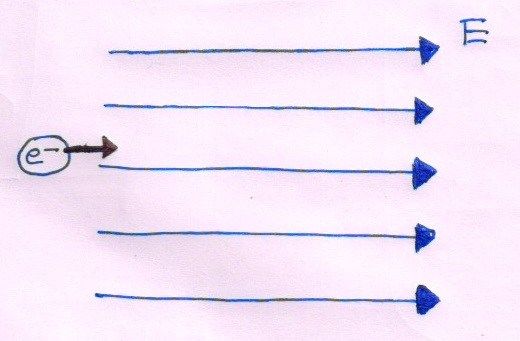# Inspired By A JEE 2014 Question

The radiation corresponding to the $2\rightarrow 1$ transition of hydrogen atom falls on a metal surface to produce photoelectrons. These electrons are made to enter an electric field of $10^{-5} \text{ N/C}$ as shown in the figure. If the electrons just change their direction of motion after 1 second, then the work function of the metal is close to which of the following choices?Details and Assumptions:

• Take the mass of electron, $m_{e}=9.1\times 10^{-31}\text{ kg}$,

• The charge on electron, $e=-1.6\times 10^{-19}\text{ C}$ and $1\text{ eV}=1.6\times 10^{-19}\text{ J}$.

• The Ionization energy of Hydrogen atom in ground state is $13.6 eV$

×